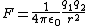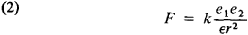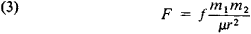# Coulomb's law

(redirected from Electrostatic interaction)
Also found in: Dictionary, Thesaurus.

## Coulomb's law

(ko͞o`lŏmz), in physics, law stating that the electrostatic force between two charged bodies is proportional to the product of the amount of charge on the bodies divided by the square of the distance between them. If the bodies are oppositely charged, one positive and one negative, they are attracted toward one another; if the bodies are similarly charged, both positive or both negative, the force between them is repulsive (see chargecharge,
property of matter that gives rise to all electrical phenomena (see electricity). The basic unit of charge, usually denoted by e, is that on the proton or the electron; that on the proton is designated as positive (+e
). Coulomb's law applies exactly only when the charged bodies are much smaller than the distance separating them and therefore can be treated approximately as point charges. When combined with principles of quantum physics, Coulomb's law helps describe the forces that bind electrons to an atomic nucleus, that bind atoms together into molecules, and that hold together solids and liquids. The law was deduced in 1785 by C. A. de CoulombCoulomb, Charles Augustin de
, 1736–1806, French physicist. In 1789 he retired from his posts as military engineer and as superintendent of waters and fountains and devoted himself to continuing his scientific research.
from experimental measures of the forces between charged bodies; the experiments were made using his torsion balance.

## Coulomb's law

For electrostatics, Coulomb's law states that the direct force F of point charge q1 on point charge q2, when the charges are separated by a distance r, is given by F = k0q1q2/r2, where k0 is a constant of proportionality whose value depends on the units used for measuring F, q, and r. It is the basic quantitative law of electrostatics. In the rationalized meter-kilogram-second (mks) system of units, k0 = 1/(4 πε0), where ε0 is called the permittivity of empty space and has the value 8.85 × 10-12 farad/m. Thus, Coulomb's law in the rationalized mks system is as in the equation below,where q1 and q2 are expressed in coulombs, r is expressed in meters, and F is given in newtons. See Electrical units and standards

The direction of F is along the line of centers of the point charges q1 and q2, and is one of attraction if the charges are opposite in sign and one of repulsion if the charges have the same sign. For a statement of Coulomb's law as applied to point magnet poles.

Experiments have shown that the exponent of r in the equation is very accurately the number 2. Lord Rutherford's experiments, in which he scattered alpha particles by atomic nuclei, showed that the equation is valid for charged particles of nuclear dimensions down to separations of about 10-12 cm. Nuclear experiments have shown that the forces between charged particles do not obey the equation for separations smaller than this. See Electrostatics

McGraw-Hill Concise Encyclopedia of Physics. © 2002 by The McGraw-Hill Companies, Inc.
The following article is from The Great Soviet Encyclopedia (1979). It might be outdated or ideologically biased.

## Coulomb's Law

one of the fundamental laws of electrostatics; determines the force of interaction between two point electric charges at rest—that is, between two electrically charged bodies whose dimensions are small compared with the distance between them. It was established experimentally in 1785 by C. Coulomb using the torsion balance, which he invented.

According to Coulomb's law, two point charges interact with each other in a vacuum with a force F whose magnitude is proportional to the product of the charges e1 and e2 and inversely proportional to the square of the distance r between them:Here k is a proportionality factor that depends on the system of units chosen: in the absolute (Gaussian) system of units (the cgs system), k = 1; in the International System of Units (SI), k = l/47T€o, where eo is the electric constant. The force F is directed along a straight line connecting the charges and is attractive for unlike charges (F < 0) and repulsive for like charges (F > 0).

If the interacting charges are situated in a homogeneous di-electric with dielectric constant €, the force of interaction is then reduced by a factor of ∊:Coulomb's law is one of the experimental foundations of classical electrodynamics; its generalization leads, among other things, to Gauss' theorem.

The law that defines the force of interaction between two magnetic poles is also called Coulomb's law:Here f is a proportionality factor (in the general case it does not coincide with k in the absolute system of units f = 1), m1 and m2 are magnetic charges, and JLI is the magnetic permeability of the medium that surrounds the interacting poles. In a vacuum F = fm1m2/r2.

### REFERENCE

Tamm, I. E. Osnovy teorii elektrichestva, 7th ed. Moscow, 1957. Chapter
1, sec. 21; ch. 2, sec. 23.

G. V. VOSKRESENSKII

## Coulomb's law

[′kü′lämz ‚lȯ]
(electricity)
The law that the attraction or repulsion between two electric charges acts along the line between them, is proportional to the product of their magnitudes, and is inversely proportional to the square of the distance between them. Also known as law of electrostatic attraction.
McGraw-Hill Dictionary of Scientific & Technical Terms, 6E, Copyright © 2003 by The McGraw-Hill Companies, Inc.
References in periodicals archive ?
Strong hypochromic effect is observed for FC-1 upon addition of ds.DNA which may be due to the electrostatic interaction between the negatively charged phosphate..
The anchoring of the dyes to the substrates was found to be compatible with either chemical binding and electrostatic interaction, or only electrostatic interaction, according to the different substituents in each rosamine.
Caption: Figure 1: Electrostatic interactions between the monomers of OKG and CH.
Then, an electrostatic interaction could be triggered with the further addition of negatively charged CpG, and the OVA-CpG NPs could self-assemble.
The response was largely suppressed in the presence of NaCl, which is attributable to disruption of the electrostatic interaction at the higher ionic strength.
This is possibly because of the electrostatic interaction between the amide (-CON[H.sub.2]) group and the MNPs.
It is of particular interest to note that for the four different binding orientations, the peptide-lipid electrostatic interaction energy overlaps with each other (see the error bar of the average value).
5; on the one hand, the range of the first shallow attractive well (where the PMF is negative) is reduced by about half when the electrostatic interaction is included, namely the range for the neutral dispersion (blue line in Fig.
The hydrophilic, cationic part is proposed to initiate electrostatic interaction with the negatively charged components (e.g., 3-9% phosphatidylserine) of the membrane of tumor cells.
In the case of the low surface areas of 184 to 353 [cm.sup.2] or SVRs between 0.7 and 1.8 [m.sup.-1], the starch was expected to first interact with the surface due to electrostatic interaction. The excess starch then has the ability to attach to the initial adsorbed layer, which is due to nonelectrostatic interactions.
In Figure 5 the effect of the screening of the interdendrimers electrostatic interaction potential is presented in water solution of sodium carboxylate terminated G3.5 PAMAM dendrimers.
Synthesis technology of the photocatalyst by utilizing the inherent electrostatic interaction between two particles

Site: Follow: Share:
Open / Close